Project showcase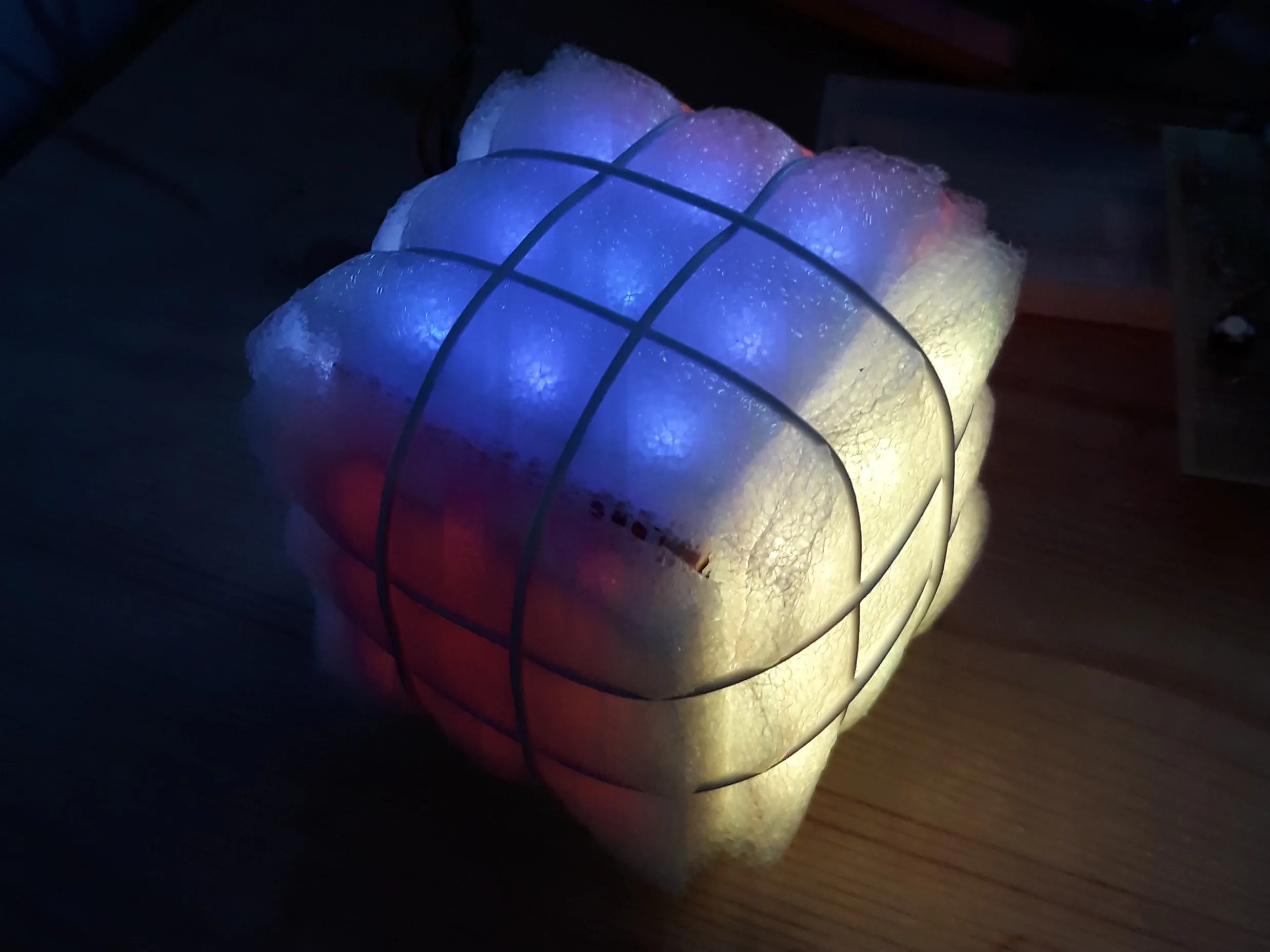Can a wooden block, wrapped in LED strips be turned into Rubik's cube?

• 3,238 views
• 14 respects

## Components and suppliesArduino UNO & Genuino UNO
×1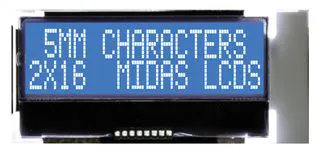Alphanumeric LCD, 16 x 2
×1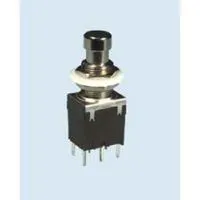Pushbutton Switch, Pushbutton
×8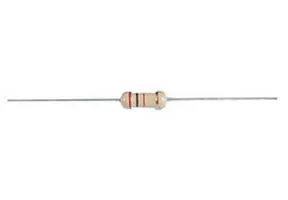Through Hole Resistor, 470 ohm
×1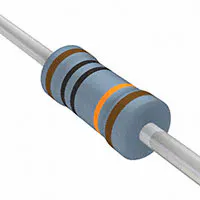Resistor 100k ohm
×2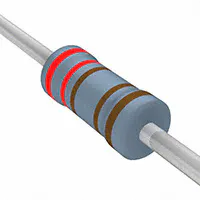Resistor 2.21k ohm
×10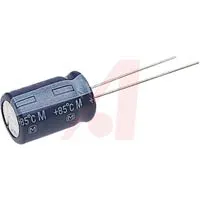Capacitor 10 µF
×1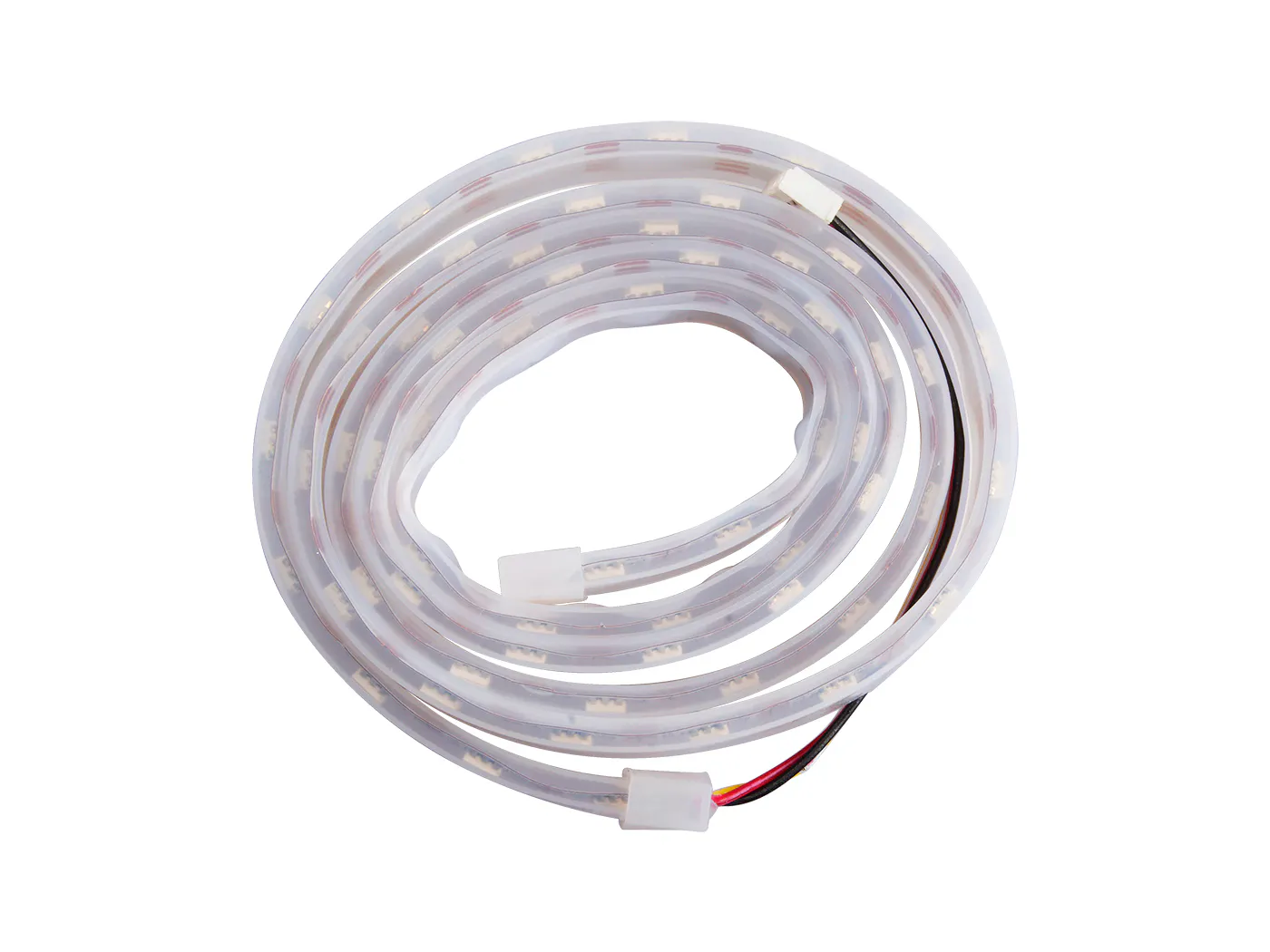Seeed Grove - WS2813 RGB LED Strip Waterproof - 60 LED/m - 1m
×1

## Necessary tools and machinesSoldering iron (generic)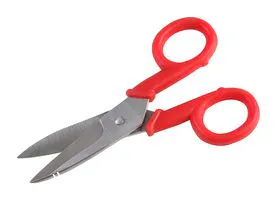Scissor, Electrician

For me this started as a challenge: Can I make a Rubik's cube without turning components?

First I made a print with push buttons for the user input. I developed a library to read this input (it reads 5+3 digital inputs using only 2 analog inputs). The user interface was completed with the standard LCD screen inside the Arduino starters package.

Then I developed the software. I started with only one side of the cube and made numbers turn around. Then I added the other sides. Now the rims needed to go together with the sides. I made a printing module that writes the representation of the cube in the memory to the serial port as an unfolded cube. This helped me with the trouble shooting.

Then the ordered LED strip arrived. The Pololu library worked fine really quickly. I started with a few LED's as I expected a lack of current from my adapter (9 * 6 sides * 3 LED's * 20 mA = 3.42 A!). It turned out that it was not at all needed to put the LED's at full brightness. The color red can be made with only the red LED at 10/256 of full brightness: Red (RGB): 10, 0, 0. So over 75 times less than the maximum power input! The same holds for blue Blue (RGB): 0, 0, 10 and Green (RGB): 0, 10, 0.

It took some experimenting to gain a red, orange and yellow that were sufficiently different. Here, it really helped to tune down the brightness of the red color. Then, orange and yellow can be made relatively much brighter. The following values gave good results: Yellow (RGB): 60, 45, 0 and Orange (RGB): 45, 15, 0. Finally I chose White (RGB): 20, 20, 20.

Now that I could make all the colours, I went on with the construction of the cube. At first I started with a 30 LED/m LED strip. I made 9 pieces of 6 LED's and 'weaved' a cube. The cube was a bit irregular and the connections were out of reach for the soldering iron. I bought a 60 LED/m LED strip and went to the toolshop where a 4.5 cm times 4.5 cm times 4.5 cm cube was made out of some residual wood for me. I adhered the LED strip around this cube and connected the wires with a soldering iron.

As my little girls cannot read English yet, I wanted a dutch version as well. Two languages caused problems as Arduino ran out of memory. Use of PROGMEM might have solved this problem, but I did not have any experience with that. Therefore, I chose to use conditional compiling for English and dutch.

Halfway the project I found out that another system of coordinates might have been better. In this alternative coordinate system quite some memory space can be saved as the rim addresses show regular patterns (see comments in code).

I thought it would be nice if the cube could automatically be solved. I implemented this by putting each move into a cube move history. If all moves in this history are turned backwards, the cube is solved again. This memory is now located in the main program. I think it would be better to put this turn history in the Cube.cpp module, but it works fine now and I decided to let it be.

Conclusions of the project:

• A wooden block wrapped in LED strips can be turned into Rubik's Cube
• It is rather easy to run out of memory
• Making nice videos of LED strips is not easy
• LED strip total current does not need to be 3.42 Amp

## Code

##### Rubik's CubeC/C++
Main program
```/* this program turns a ledstrip glued to a wooden cube into a Rubiks Cube
*
*/
//major changes on March 18th 2019
#include "choices.h"
#include "cube.h"
#include "output.h"
#include <LiquidCrystal.h>
#include <PololuLedStrip.h>

Choices LeftPanel(A5, 5); //set 5 options at pin A5
Choices RightPanel(A4, 3);//set 3 options at pin A3
Cube MyCube(3);           //would be nice to have DO port of ledstrip as an argument
Output MyOutput(false, true);  //output to Serial output and or lcd output

#define _dutch   //dutch language selected, conditional compiling is applied because insufficient dynamic memory to store 2 languages

void setup() {
Serial.begin(9600);
delay(500);
MyCube.show();
delay(500);
MyOutput.clrscr();
#ifndef _dutch
MyOutput.txt("Hello there!");  //default language
#else
MyOutput.txt("Hallo daar!");
#endif
MyCube.littleShow();
MyOutput.clrscr();
}

const int turnSide  = 0;
const int turnMid   = 1;
const int turnCube  = 2;
const int undoLast  = 3;
const int solveCube = 4;

const bool CW = true;

const int histMax = 30;    //max number of turns in memory
int turnNr      = 0;       //number of turns done
int lastSolved  = 0;       //last time that cube was solved
int turnsInHist = 0;       //current number of turns in history
byte turnHist[histMax];
/* turns are stored in turnHist
*  1 byte is used to store turn
*  1 byte may represent numbers from 0 - 255
*  coding: 100*dir + 10 * side + task
*  history should be implemented in Class Cube
*/
bool escFlag = false;

void loop() {

int side;
bool dir;

escFlag = false;
case turnSide:
while (true) {
side = getSide();
if (escFlag) break;
dir  = getDir();
if (escFlag) break;
MyCube.showTurnSide(side, dir);
turnNr++;
turnsInHist++;
}
break;
case turnMid:
while (true) {
side = getSide();
if (escFlag) break;
dir  = getDir();
if (escFlag) break;
MyCube.showTurnMid(side, dir);
turnNr++;
turnsInHist++;
}
break;
case turnCube:
while (true) {
side = getSide();
if (escFlag) break;
dir  = getDir();
if (escFlag) break;
MyCube.showTurnCube(side,dir);
turnNr++;
turnsInHist++;
}
break;
case undoLast:
do {
if (turnsInHist == 0) {
MyOutput.clrscr();
#ifndef _dutch
MyOutput.txt("Cannot undo");
#else
MyOutput.txt("Ongedaan maken");
MyOutput.nxtln();
MyOutput.txt("niet mogelijk");
#endif
delay(1000);
escFlag = true;
}
if (escFlag) break;
turnNr--;
turnsInHist--;
undoLastTurn();
delay(500);
if (turnsInHist == 0) break;
MyOutput.clrscr();
#ifndef _dutch
MyOutput.txt("Undo another");
MyOutput.nxtln();
MyOutput.txt("turn?");
#else
MyOutput.txt("Nogmaals");
MyOutput.nxtln();
MyOutput.txt("terug draaien?");
#endif
while (!escFlag) {
int choice = RightPanel.getOption();
if (choice == RightPanel.highBut) {
escFlag = true;
break;
}
if (choice == RightPanel.lowBut) {
break;
}
}
} while (!escFlag);
break;
case solveCube:
if ((turnsInHist == 0) || ((turnNr-lastSolved)>turnsInHist)) {  //not enough memory to undo until solved
MyCube.reInit();
lastSolved = 0;
turnNr = 0;
turnsInHist = 0;
}
else {
while(turnNr > lastSolved) {
turnNr--;
turnsInHist--;
undoLastTurn();
delay(500);
}
}
break;
}
if (MyCube.checkCubeSolved()) {
MyOutput.clrscr();
#ifndef _dutch
MyOutput.txt("Cube solved!");
#else
MyOutput.txt("Kubus opgelost!");
#endif
for (int i=0; i<5; i++) {
delay(500);
MyCube.dark();
delay(500);
MyCube.show();
lastSolved = turnNr;
}
}
if (turnsInHist>histMax) turnsInHist = histMax;
delay(500);
}

void undoLastTurn() {
byte dir = turnHist[turnNr%histMax]/100;
byte side = (turnHist[turnNr%histMax]-100*dir)/10;
MyCube.showTurnSide(side,!dir);
}
MyCube.showTurnMid(side,!dir);
}
MyCube.showTurnCube(side,!dir);
}
}

#ifndef _dutch
const String Tasks[] = {"Turn side ",
"Turn mids ",
"Turn cube ",
"Undo turn ",
"Solve cube"};
#else
const String Tasks[] = {"Draai zijkant",
"Draai midden ",
"Draai kubus  ",
"Draai terug  ",
"Los kubus op "};
#endif

MyOutput.clrscr();
#ifndef _dutch
#else
MyOutput.txt("Kies taak>");
#endif
MyOutput.nxtln();
int ans = turnSide;
MyOutput.nxtln();
while (!confirmed()) {
int old_ans = ans;
int userInput = LeftPanel.getOption();
if (userInput == LeftPanel.arrowUp   || userInput == LeftPanel.arrowRight)   ans++;
if (userInput == LeftPanel.arrowDown || userInput == LeftPanel.arrowLeft) ans--;
if (ans==-1)  ans = solveCube;
if (ans== 5)  ans = turnSide;
if (ans != old_ans) {
MyOutput.nxtln();
delay(100);
}
}
MyOutput.nxtln();
MyOutput.clrscr();
#ifndef _dutch
MyOutput.txt("Choice= ");
#else
MyOutput.txt("Keuze= ");
#endif
MyOutput.nxtln();
delay(1000);
return ans;
}

int getSide() {

#ifndef _dutch
const String Txts[]       = {"Turn ",
" side"};
const String SidesTxt[]  = {"ground",
"front ",
" top  ",
" back ",
" left ",
"right "};

#else
const String Txts[]       = {"Draai ",
"kant"};

const String SidesTxt[]  = {" onder",
"  voor",
" boven",
"achter",
"rechter"};
#endif

MyOutput.nxtln();
MyOutput.clrscr();
#ifndef _dutch
MyOutput.txt("Choose side/axis>");
#else
MyOutput.txt("Kies zijkant/as>");
#endif
MyOutput.nxtln();
MyOutput.txt(Txts);
int ans = MyCube.frontSide;
MyOutput.txt(SidesTxt[ans]);
MyOutput.txt(Txts);

while (!confirmed()) {
if (RightPanel.getOption() == RightPanel.highBut) {
escFlag = true;
return;
}
int old_ans = ans;
int userInput = LeftPanel.getOption();
if (ans > -1 && ans < 4) {
if (userInput == LeftPanel.arrowUp) {
ans++;
if (ans == 4) {
ans = MyCube.groundSide;
}
}
if (userInput == LeftPanel.arrowDown) {
ans--;
if (ans == -1) {
ans = MyCube.backSide;
}
}
if (userInput == LeftPanel.arrowLeft) {
ans = MyCube.leftSide;
}
if (userInput == LeftPanel.arrowRight) {
ans = MyCube.rightSide;
}
}
if (ans == MyCube.leftSide) {
if (userInput == LeftPanel.arrowRight) {
ans = MyCube.frontSide;
}
if (userInput == LeftPanel.arrowUp) {
ans = MyCube.topSide;
}
if (userInput == LeftPanel.arrowDown) {
ans = MyCube.groundSide;
}
}
if (ans == MyCube.rightSide) {
if (userInput == LeftPanel.arrowLeft) {
ans = MyCube.frontSide;
}
if (userInput == LeftPanel.arrowUp) {
ans = MyCube.topSide;
}
if (userInput == LeftPanel.arrowDown) {
ans = MyCube.groundSide;
}
}
if (userInput == LeftPanel.arrowCentre) {
ans = MyCube.frontSide;
}
if (ans != old_ans) {
MyOutput.nxtln();
MyOutput.txt(Txts);
MyOutput.txt(SidesTxt[ans]);
MyOutput.txt(Txts);
delay(100);
}
}
MyOutput.clrscr();
#ifndef _dutch
MyOutput.txt("Choice= ");
#else
MyOutput.txt("Keuze = ");
#endif
MyOutput.nxtln();
MyOutput.txt(Txts);
MyOutput.txt(SidesTxt[ans]);
MyOutput.txt(Txts);
delay(1000);
return ans;
}

bool getDir() {

#ifndef _dutch
const String DirTxt[]   = {"Left  turn",
"Right turn"};
#else
const String DirTxt[]   = {"Linksom ",
"Rechtsom"};
#endif
bool CW = 1;
bool ans = CW;

MyOutput.clrscr();
#ifndef _dutch
MyOutput.txt("Choose direction>");
#else
MyOutput.txt("Kies richting>");
#endif
MyOutput.nxtln();
MyOutput.txt(DirTxt[ans]);
while (!confirmed()) {
if (RightPanel.getOption() == RightPanel.highBut) {
escFlag = true;
return;
}
bool old_ans = ans;
int userInput = LeftPanel.getOption();
if (userInput != 0) {
ans = !ans;
}
if (ans != old_ans) {
MyOutput.nxtln();
MyOutput.txt(DirTxt[ans]);
delay(100);
}
}
MyOutput.clrscr();
#ifndef _dutch
MyOutput.txt("Direction=");
#else
MyOutput.txt("Richting=");
#endif
MyOutput.nxtln();
MyOutput.txt(DirTxt[ans]);
delay(1000);
return ans;
}

bool confirmed() {
int UserInput = RightPanel.getOption();
if (UserInput == RightPanel.lowBut) {
return 1;
}
return 0;
}
```
##### cube.hC/C++
Cube: Library that represents and manipulates Cube
```/*
Cube.h - Library for Rubik's Cube
Created by Koen Meesters, March 5th 2019
Last edited by Koen Meesters,
*/
#ifndef cube_h
#define cube_h

#include "Arduino.h"
#include  <PololuLedStrip.h>

class Cube
{
public:
Cube(int);
int noIdeaWhy;                      //somehow a class cannot have a constructor without an arguement...
void reInit();                      //reinitializes the Cube
void show();
void dark();
void showTurnSide(int side,bool dir);
void showTurnMid(int side,bool dir);
void showTurnCube(int side,bool dir);
bool checkCubeSolved();             //checks if the cube is solved
void littleShow();                  //gives a little show
void toScreen();                    //writes Cube to computer screen via Serial
const byte* handCube();             //hands Cube to main program

static const int numSides = 6;      //Cube has 6 sides

const int backSide   = 3;
const int topSide    = 2;
const int frontSide  = 1;
const int groundSide = 0;
const int leftSide   = 4;
const int rightSide  = 5;

static const int numPos  = 9;        //Each side has 9 positions  //needs to be static zijn as number of array elements needs to be fixed before compilation

const bool CW = true;

private:

void turnSide(int, bool);           //turns a side of Cube
void turnRim(int, bool);            //turns rim of a side of Cube
void turnMid(int, bool);            //turns the mids of of Cube
int _noIdeaWhy;
byte Color[numSides][numPos];         //dach position has a color that will be represented in a byte
static const int numRimPos=12;        //dach rim has 12 positions
static const int numMidPos=12;        //each mid has 12 positions
byte* rimsPtr[numSides][numRimPos];   //array of pointers to addresses of the rim positions of a Side, used in turnRim()
byte* midsPtr[numSides][numRimPos];   //array of pointers to addresses of the mids positions of a Side, used in turnMid()
byte delta_t = 150;                   //gives good visuals
};
#endif
```
##### cube.cppC/C++
Cube: Library that represents and manipulates Cube
```/*
Cube.h - Library for Rubik's Cube
Created by Koen Meesters, March 5th 2019
Last edited by Koen Meesters,
*/
#define LED_COUNT 54

#include "Arduino.h"
#include "cube.h"
#include <PololuLedStrip.h>

PololuLedStrip<2> ledStrip;  //Sturing ledstrip op DO 2

Cube::Cube(int) {

int _noIdeaWhy=noIdeaWhy;

//initialize cube
for (int curSide = 0; curSide < numSides; curSide++) {    //fill all sides
for (int curPos = 0; curPos < numPos; curPos++) {       //fill all positions
Color[curSide][curPos] = 10*curSide + curPos;
}
}

//initialize Rims        01    02    03    04    05    06    07    08    09    10    11    12
byte rims = {{{1,7},{1,6},{1,5},{5,7},{5,6},{5,5},{3,3},{3,2},{3,1},{4,7},{4,6},{4,5}}, //rims side 0
{{2,7},{2,6},{2,5},{5,1},{5,8},{5,7},{0,3},{0,2},{0,1},{4,5},{4,4},{4,3}}, //rims side 1
{{3,7},{3,6},{3,5},{5,3},{5,2},{5,1},{1,3},{1,2},{1,1},{4,3},{4,2},{4,1}}, //rims side 2
{{0,7},{0,6},{0,5},{5,5},{5,4},{5,3},{2,3},{2,2},{2,1},{4,1},{4,8},{4,7}}, //rims side 3

{{2,1},{2,8},{2,7},{1,1},{1,8},{1,7},{0,1},{0,8},{0,7},{3,1},{3,8},{3,7}}, //rims side 4
{{2,5},{2,4},{2,3},{3,5},{3,4},{3,3},{0,5},{0,4},{0,3},{1,5},{1,4},{1,3}}};//rims side 5

for (int curSide = 0; curSide < numSides; curSide++) {
for (int curRimPos = 0; curRimPos < numRimPos; curRimPos++) {
const int side=0;  //element index of Side in rims
const int pos=1;   //element index of Position in rims
rimsPtr[curSide][curRimPos] = &Color[rims[curSide][curRimPos][side]][rims[curSide][curRimPos][pos]];
}
}

//intitialize Mids       01    02    03    04    05    06    07    08    09    10    11    12
byte mids = {{{1,8},{1,0},{1,4},{5,8},{5,0},{5,4},{3,4},{3,0},{3,8},{4,8},{4,0},{4,4}}, //mids side 0
{{2,8},{2,0},{2,4},{5,2},{5,0},{5,6},{0,4},{0,0},{0,8},{4,6},{4,0},{4,2}}, //mids side 1
{{3,8},{3,0},{3,4},{5,4},{5,0},{5,8},{1,4},{1,0},{1,8},{4,4},{4,0},{4,8}}, //mids side 2
{{0,8},{0,0},{0,4},{5,6},{5,0},{5,2},{2,4},{2,0},{2,8},{4,2},{4,0},{4,6}}, //mids side 3

{{2,2},{2,0},{2,6},{1,2},{1,0},{1,6},{0,2},{0,0},{0,6},{3,2},{3,0},{3,6}}, //mids side 4
{{2,6},{2,0},{2,2},{3,6},{3,0},{3,2},{0,6},{0,0},{0,2},{1,6},{1,0},{1,2}}};//mids side 5

for (int curSide = 0; curSide < numSides; curSide++) {
for (int curMidPos = 0; curMidPos < numMidPos; curMidPos++) {
const int side=0;  //element index of Side in mids
const int pos=1;   //element index of Position in mids
midsPtr[curSide][curMidPos] = &Color[mids[curSide][curMidPos][side]][mids[curSide][curMidPos][pos]];
}
}
}
/*
Each side has 9 positions
[ 1][ 2][ 3]
[ 8][ 0][ 4]
[ 7][ 6][ 5]

6 sides together form a cube                                  Ledstripn addresses
0 ground
  
  
  
3 Back                                             3 back
[ 1][ 2][ 3]                                         
[ 8][ 0][ 4]                                         
[ 7][ 6][ 5]                                         
2 Top                                              2 Top
[ 1][ 2][ 3]                                         
[ 8][ 0][ 4]                                         
[ 7][ 6][ 5]                                         
4 Left       1 Front      5 Right                4 Left        1 Front         5 Right
[ 1][ 2][ 3] [ 1][ 2][ 3] [ 1][ 2][ 3]           [ 2] [ 3] [ 8]      
[ 8][ 0][ 4] [ 8][ 0][ 4] [ 8][ 0][ 4]           [ 1] [ 4] [ 7]      
[ 7][ 6][ 5] [ 7][ 6][ 5] [ 7][ 6][ 5]           [ 0] [ 5] [ 6] [ 9]     
0 Ground
[ 1][ 2][ 3]
[ 8][ 0][ 4]
[ 7][ 6][ 5]

//vertaaltqbel cube naar Ledstrip
31,20,32,44,43,42,30,18,19,
22,11,23,35,34,33,21, 9,10,
25,14,26,38,37,36,24,12,13,
28,17,29,41,40,39,27,15,16,
4, 2, 3, 8, 7, 6, 5, 0, 1,
49,53,48,47,46,45,50,51,52

Each side has a number and a character ID
0 = groundSide
1 = frontSide
2 = topSide
3 = backSide
4 = leftSide
5 = rightSide

The cube will have 6 * 9 = 54 RGB LEDS, so 162 LED's!
*/

//This function checks if the cube is solved (each side has one color)
bool Cube::checkCubeSolved() {
for (int curSide = 0; curSide < numSides; curSide++) {    //check all sides
byte sideColor = Color[curSide]/10;
for (int curPos = 1; curPos < numPos; curPos++) {       //fill all positions
if (!(Color[curSide][curPos]/10==sideColor)) return false;
}
}
return true;
}

//This procedure reinitializes the cube
void Cube::reInit() {
for (int curSide = 0; curSide < numSides; curSide++) {    //fill all sides
for (int curPos = 0; curPos < numPos; curPos++) {       //fill all positions
Color[curSide][curPos] = 10*curSide + curPos;       //later colours will be set here
}
}
show();
}

//This procedure does a little show
void Cube::littleShow() {
delay(1000);
for (int i=0; i<4; i++) {
showTurnMid(i,!CW);
delay(1000);
}
for (int i=3; i>=0; i--) {
showTurnMid(i,CW);
delay(1000);
}
delay(1000);
reInit();
}

//This procedure shows a turn of the cube as a whole
void Cube::showTurnCube(int side,bool dir) {
showTurnSide(side,dir);
showTurnMid(side,dir);
int oppSide = 0;
if (side<2) oppSide = side+2;
if (side>1 && side<4) oppSide = side-2;
if (side==4) oppSide = 5;
if (side==5) oppSide = 4;
showTurnSide(oppSide,!dir);
delay(delta_t);
}

//This procedure shows a turn of a side
void Cube::showTurnSide(int side,bool dir) {
turnRim(side,dir);
show();
delay(delta_t);
for (int i = 0; i<2; i++) {
turnSide(side,dir);
show();
delay(delta_t);
turnRim(side,dir);
show();
delay(delta_t);
}
}

//This procedrue shows a turn of the mids
void Cube::showTurnMid(int side,bool dir) {
for (int i = 0; i<3; i++) {
turnMid(side,dir);
show();
delay(delta_t);
}
}

//This procedure turns the side
void Cube::turnSide(int side, bool dir) {                   //this procedure must be run 2 times per turn
byte tempStoreColor;
if (dir == CW) {                                          //turn ClockWise
tempStoreColor = Color[side][numPos-1];                 //Color value of Position 8 is temporarily stored
for (int curPos = numPos-2; curPos > 0; curPos--) {
Color[side][curPos+1] = Color[side][curPos];          //shift Color value Clockwise
}
Color[side] = tempStoreColor;                        //put stored Color value in Position 1
}
else {                                                    //turn Counter ClockWise
tempStoreColor = Color[side];                        //Color value of Position 1 is temporarily stored
for (int curPos = 1; curPos < numPos-1; curPos++) {
Color[side][curPos] = Color[side][curPos+1];          //shift Color value counter Clockwise
}
Color[side][numPos-1] = tempStoreColor;                 //put stored Color value in Position 8
}
}

//this procedure turns de mids
void Cube::turnMid(int side, bool dir) {                        //this procedure must be run 3 times per turn
byte tempStoreColor;
if (dir == CW) {                                              //if turn ClockWise
tempStoreColor = *midsPtr[side][numRimPos-1];               //Color value of Position 12 is temporarily stored
for (int curPos = numMidPos-1; curPos > 0; curPos--) {
*midsPtr[side][curPos] = *midsPtr[side][curPos-1];        //shift Color value Clockwise
}
*midsPtr[side] = tempStoreColor;                         //put stored Color value in Position 0
}
else {                                                        //turn Counter ClockWise
tempStoreColor = *midsPtr[side];                         //Color value of Position 0 is temporarily stored
for (int curPos = 0; curPos < numMidPos-1; curPos++) {
*midsPtr[side][curPos] = *midsPtr[side][curPos+1];        //shift Color value Clockwise
}
*midsPtr[side][numRimPos-1] = tempStoreColor;               //put stored Color value in Position 12
}
}

//This procedure turns the rims of a Side
void Cube::turnRim(int side, bool dir) {                       //this procedure must be run 3 times per turn
byte tempStoreColor;
if (dir == CW) {                                              //turn ClockWise
tempStoreColor = *rimsPtr[side][numRimPos-1];               //Color value of Position 12 is temporarily stored
for (int curPos = numRimPos-1; curPos > 0; curPos--) {
*rimsPtr[side][curPos] = *rimsPtr[side][curPos-1];        //shift Color value Clockwise
}
*rimsPtr[side] = tempStoreColor;                         //put stored Color value in Position 0
}
else {                                                        //turn counter clockwise
tempStoreColor = *rimsPtr[side];                         //Color value of Position 0 is temporarily stored
for (int curPos = 0; curPos < numRimPos-1; curPos++) {
*rimsPtr[side][curPos] = *rimsPtr[side][curPos+1];        //shift Color value counter clockwise
}
*rimsPtr[side][numRimPos-1] = tempStoreColor;               //put stored Color value in Position 12
}
}

//This function hands the address of the cube to the ledstrip writing procedure
const byte* Cube::handCube() {
return &Color;
}

//#define _debugging
#ifdef _debugging
//This procedure writes the Cube colors to Serial (for debugging purposes)
void Cube::toScreen() {
Serial.println("Cube");
int transPos[] = { 1,2,3,
8,0,4,
7,6,5 };
int curSide;

//backSide and TopSide
for (curSide = backSide; curSide >= topSide; curSide--) {
for (int i=0; i<3; i++) { // voor alle posities
Serial.print("                ");
for (int j=(3*i); j<(3+3*i); j++) {
int pos = transPos[j];
Serial.print('[');
if(Color[curSide][pos]<9) {Serial.print(' ');}
Serial.print(Color[curSide][pos]);
Serial.print("] ");
}
Serial.println();
}
Serial.println();
}

//leftSide, frontSide and rightSide
for (int i=0; i<3; i++) { // voor alle posities
curSide = leftSide;
for (int j=(3*i); j<(3+3*i); j++) {
int pos = transPos[j];
Serial.print('[');
if(Color[curSide][pos]<9) {Serial.print(' ');}
Serial.print(Color[curSide][pos]);
Serial.print("] ");
}
Serial.print(' ');
curSide = frontSide;
for (int j=(3*i); j<(3+3*i); j++) {
int pos = transPos[j];
Serial.print('[');
if(Color[curSide][pos]<9) {Serial.print(' ');}
Serial.print(Color[curSide][pos]);
Serial.print("] ");
}
Serial.print(' ');
curSide = rightSide;
for (int j=(3*i); j<(3+3*i); j++) {
int pos = transPos[j];
Serial.print('[');
if(Color[curSide][pos]<9) {Serial.print(' ');}
Serial.print(Color[curSide][pos]);
Serial.print("] ");
}
Serial.println();
}
Serial.println();

//groundSide
curSide = groundSide;
for (int i=0; i<3; i++) { // voor alle posities
Serial.print("                ");
for (int j=(3*i); j<(3+3*i); j++) {
int pos = transPos[j];
Serial.print('[');
if(Color[curSide][pos]<9) {Serial.print(' ');}
Serial.print(Color[curSide][pos]);
Serial.print("] ");
}
Serial.println();
}
Serial.println();
}
#endif

/* alternative Cube config
*
*                        51 52 53
*                        58 50 54
*                        57 56 55
*
*              31 32 33  41 42 43
*              38 30 34  48 40 44
*              37 36 35  47 46 45
*
*    11 12 13  21 22 23
*    18 10 14  28 20 24
*    17 16 15  27 26 25
*
*    01 02 03
*    08 00 04
*    07 06 05
*
*rims
*0:    17 16 15  27 26 25
*1:    31 38 37  21 28 27  03 02 01
*2:    37 36 35  47 46 45  05 04 03  15 14 13
*3:    51 58 57  41 48 47  23 22 21  13 12 11
*4:    57 56 55            25 24 23  35 34 33
*5:                        43 42 41  33 32 31
*
*mids
*0:    18 10 14  28 20 24
*1:    32 30 36  22 20 26  04 00 08
*2:    38 30 34  48 40 44  06 00 02  16 10 12
*3:    52 50 56  42 40 46  24 20 28  14 10 18
*4:    58 50 54            26 20 22  36 30 32
*5:                        44 40 48  34 30 38
*
*
*/

void Cube::dark() {
rgb_color Dark;
Dark.red     =  0;
Dark.green   =  0;
Dark.blue    =  0;

rgb_color colors;
for (int i=0; i<54; i++) {
colors[i] = Dark;
}
ledStrip.write(colors, LED_COUNT);
}

void Cube::show() {

rgb_color Red;
Red.red      = 10;
Red.green    =  0;
Red.blue     =  0;

rgb_color Blue;
Blue.red     =  0;
Blue.green   =  0;
Blue.blue    = 10;

rgb_color Green;
Green.red    =  0;
Green.green  = 10;
Green.blue   =  0;

rgb_color Yellow;
Yellow.red   = 60;
Yellow.green = 45;
Yellow.blue  =  0;

rgb_color White;
White.red    = 20;
White.green  = 20;
White.blue   = 20;

rgb_color Orange;
Orange.red   = 45;
Orange.green = 15;
Orange.blue  =  0;

rgb_color Dark;
Dark.red     =  0;
Dark.green   =  0;
Dark.blue    =  0;

rgb_color Colors;

22,11,23,35,34,33,21, 9,10,
25,14,26,38,37,36,24,12,13,
28,17,29,41,40,39,27,15,16,
4, 2, 3, 8, 7, 6, 5, 0, 1,
49,53,48,47,46,45,50,51,52};

for (int i=0; i<54; i++) {
Colors[i] = Dark;
}

const byte* cubePtr = handCube();
for (int i = 0; i<54; i++) {

byte curColor = *cubePtr/10;
switch (curColor) {
case 0:
break;
case 1:
break;
case 2:
break;
case 3:
break;
case 4:
break;
case 5:
break;
}
cubePtr++;
}
ledStrip.write(Colors, LED_COUNT);
}

```
##### choices.hC/C++
```/*
Created by Koen Meesters, February 21st 2019
Last edited by Koen Meesters, February 21st 2019
*/
#ifndef choices_h
#define choices_h

#include "Arduino.h"

class Choices
{
public:
Choices(int AIpin, int numOpt);
int getOption();
const int arrowUp     = 1;
const int arrowLeft   = 2;
const int arrowCentre = 3;
const int arrowRight  = 4;
const int arrowDown   = 5;
const int highBut     = 1;
const int midBut      = 2;
const int lowBut      = 3;

private:
int _AIpin;
int _numOpt;
};

#endif
```
##### choices.cppC/C++
```/*
Created by Koen Meesters, February 21st 2019
Last edited by Koen Meesters, February 21st 2019
*/

#include "Arduino.h"
#include "choices.h"

Choices::Choices(int AIpin, int numOpt)
{
_AIpin = AIpin;
_numOpt = numOpt;
}

int Choices::getOption() {
bool valid = 0;

while (!valid) {

const int maxValue = 1024;
int stepSize = maxValue/(_numOpt+1);
int validZone = stepSize/4;

for (int curOpt = 0; curOpt <= _numOpt; curOpt++) {
if (value > curOpt*stepSize-validZone && value < curOpt*stepSize+validZone) {
return(curOpt);
}
}
}
}

const int numReads = 10; //Value is measured multiple times
analogReference(EXTERNAL);
int totVal  = 0;
int lowest  = 1024;
int highest = 0;
delay(10);
for (int i = 0; i < numReads; i++) {
if (curVal < lowest) lowest = curVal;
if (curVal > highest) highest = curVal;
totVal += curVal;
delay(10);
}
if ((highest - lowest)>50) value = 0;
return(value);
}
```
##### output.hC/C++
Writes output to LCD and serial in one go (mainly handy for trouble shooting)
```/*
output.h - Library to handle serial and lcd screen output
Created by Koen Meesters, March 2019
Last edited by Koen Meesters, April 13th 2019
*/
#ifndef output_h
#define output_h

#include "Arduino.h"
#include <LiquidCrystal.h>

class Output
{
public:
Output(bool serial,bool cryst);
int noIdeaWhy;
void txt(String);
void nxtln();
void clrscr();
private:
bool _serial;
bool _cryst;
};

#endif
```
##### output.cppC/C++
Writes output to LCD and serial in one go (mainly handy for trouble shooting)
```/*
output.cpp - Library to handle serial and lcd screen output
Created by Koen Meesters, March 2019
Last edited by Koen Meesters, April 13th 2019
*/

#include "Arduino.h"
#include "output.h"

LiquidCrystal lcd(8, 9, 10, 11, 12, 13);

Output::Output(bool serial, bool cryst) {
lcd.begin(16,2);
_serial = serial;
_cryst  = cryst;
}

void Output::clrscr() {          //clear screen (go to next line in Serial output)
if (_serial) {
Serial.println();
}
if (_cryst) {
lcd.clear();
}
}

void Output::txt(String text) {   //write text
if (_serial) {
Serial.print(text);
}
if (_cryst) {
lcd.print(text);
}
}

void Output::nxtln() {             //go to next line
if (_serial) {
Serial.println();
}
if (_cryst) {
lcd.setCursor(0,1);
}
}
```

## Schematics

#### Author

• Pololu led strip control library by Pololu

June 26, 2019

#### Members who respect this project

See similar projects
you might like

#### Cube for Kids

Project tutorial by Thomas Angielsky

• 2,271 views
• 9 respects

#### Interactive 4x4x4 LED cube

Project tutorial by Tong Xin Hua

• 3,658 views
• 1 respect

#### LED Cube 7x7x7

Project tutorial by Marcazzan_M

• 12,237 views
• 52 respects

• 11,438 views
• 18 respects

#### RGB LED Cube With Bluetooth App + AnimationCreator

Project showcase by PhilKey

• 4,143 views
• 1 comment
• 24 respects

#### LED CUBE 4x4x4

Project showcase by MakerRobotics

• 43,392 views